#2
14
01. 云栖社区>
2. 博客>
3. 正文

## 教你打造股市晴雨表——通过LSTM神经网络预测股市

【方向】 2017-01-08 20:01:54 浏览36287

Jakob Aungiers 现就职于汇丰银行伦敦总部，担任全球资产管理的开发副总裁。擅长机器学习，神经网络等领域。(以下为译文)

(用作训练/测试文件的链接在这里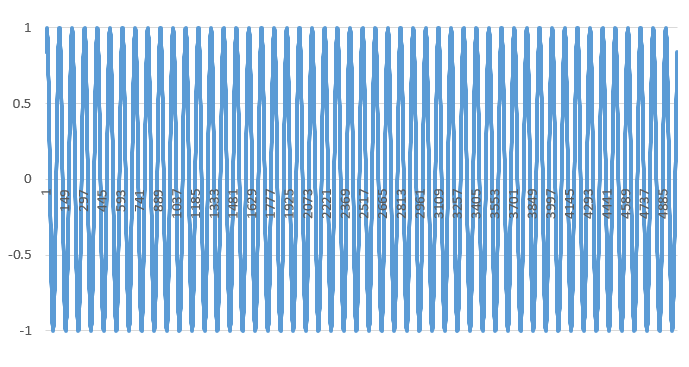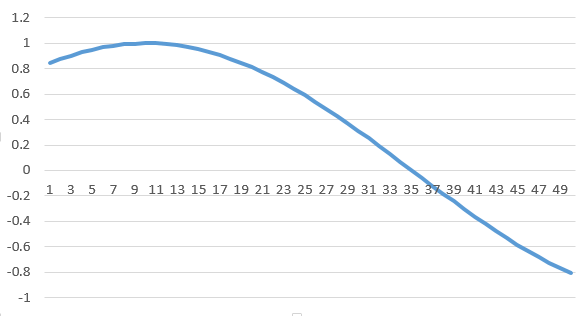def load_data(filename):
f = open(&#39;sinwave.csv&#39;, &#39;rb&#39;).read()
data = f.split(&#39;\r\n&#39;)

sequence_length = 50
result = []
for index in range(len(data) - sequence_length):
result.append(data[index: index + sequence_length])

result = np.array(result)

row = round(0.9 * result.shape)
train = result[:row, :]
np.random.shuffle(train)
x_train = train[:, :-1]
y_train = train[:, -1]
x_test = result[row:, :-1]
y_test = result[row:, -1]

x_train = np.reshape(x_train, (x_train.shape, x_train.shape, 1))
x_test = np.reshape(x_test, (x_test.shape, x_test.shape, 1))

return [x_train, y_train, x_test, y_test]

def build_model(layers):
model = Sequential()

input_dim=layers,
output_dim=layers,
return_sequences=True))

layers,
return_sequences=False))

output_dim=layers))

start = time.time()
model.compile(loss=&quot;mse&quot;, optimizer=&quot;rmsprop&quot;)
print &quot;Compilation Time : &quot;, time.time() - start
return model

epochs  = 1seq_len = 50

X_train, y_train, X_test, y_test = lstm.load_data('sinwave.csv')

model = lstm.build_model([1, 50, 100, 1])

model.fit(

X_train,
y_train,
batch_size=512,
nb_epoch=epochs,
validation_split=0.05)


predicted = lstm.predict_point_by_point(model, X_test)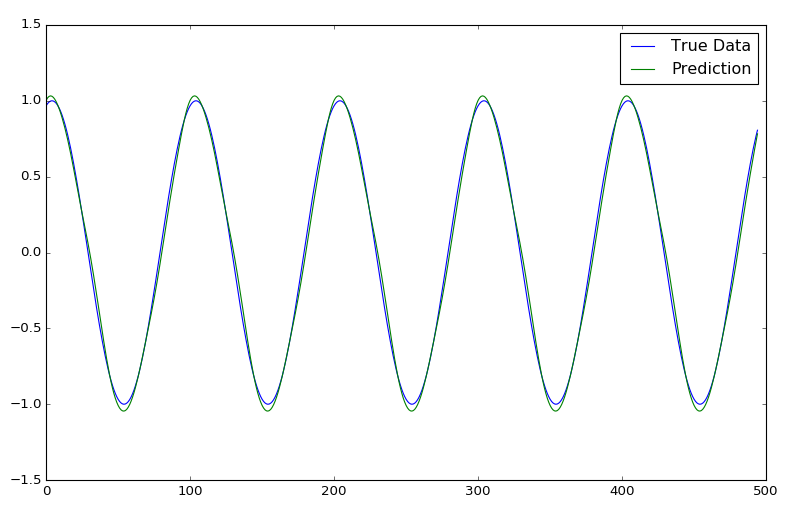epochs = 1, window size = 50epochs = 1, window size = 50

n =价格变化的标准化列表[窗口]
p =原始列表[窗口]调整的每日回报价格

-1)

)

def load_data(filename, seq_len, normalise_window):
f = open(filename, &#39;rb&#39;).read()
data = f.split(&#39;\r\n&#39;)

sequence_length = seq_len + 1
result = []
for index in range(len(data) - sequence_length):
result.append(data[index: index + sequence_length])

if normalise_window:
result = normalise_windows(result)

result = np.array(result)

row = round(0.9 * result.shape)
train = result[:row, :]
np.random.shuffle(train)
x_train = train[:, :-1]
y_train = train[:, -1]
x_test = result[row:, :-1]
y_test = result[row:, -1]

x_train = np.reshape(x_train, (x_train.shape, x_train.shape, 1))
x_test = np.reshape(x_test, (x_test.shape, x_test.shape, 1))

return [x_train, y_train, x_test, y_test]


def normalise_windows(window_data):

normalised_data = []
for window in window_data:
normalised_window = [((float(p) / float(window)) - 1) for p in window]
normalised_data.append(normalised_window)
return normalised_data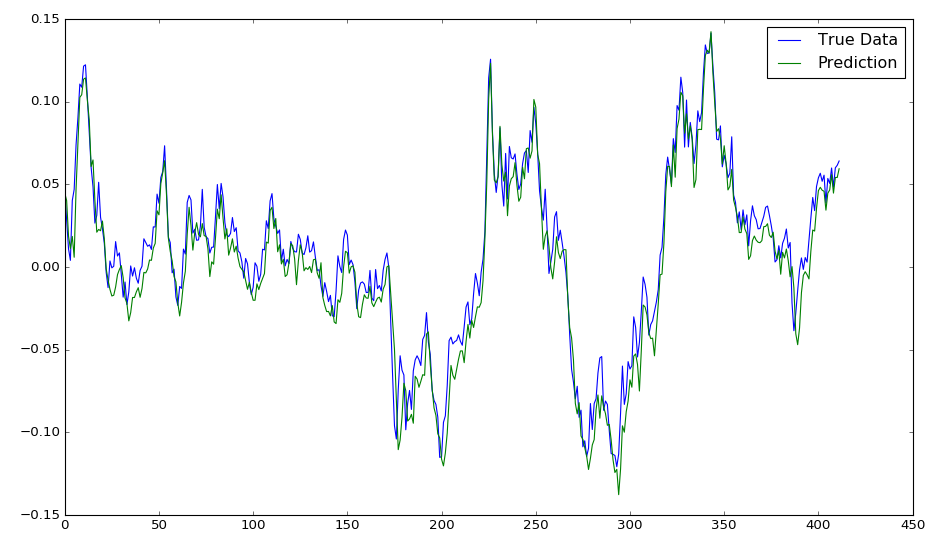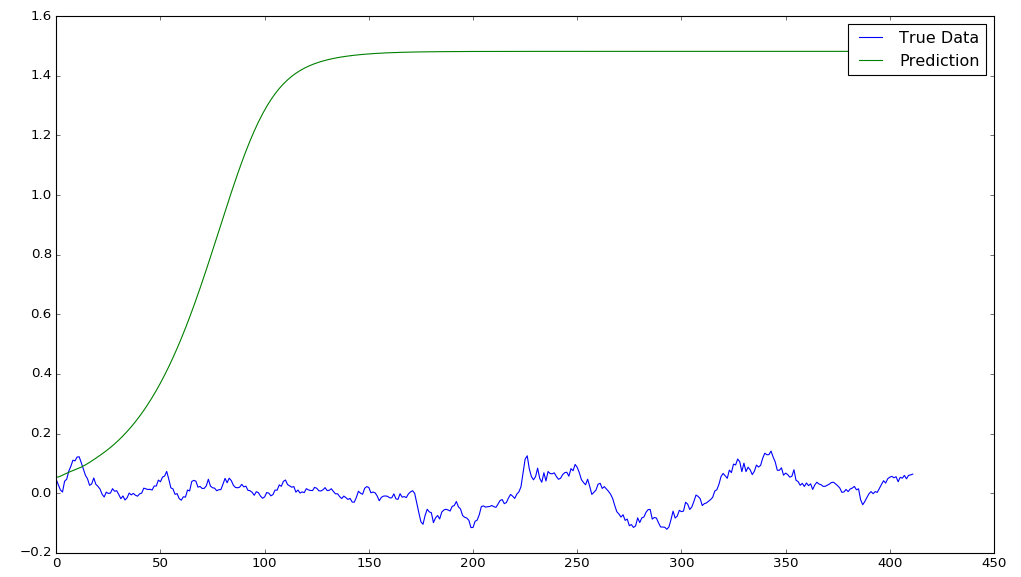epochs = 50, window size = 50epochs = 1, window size = 50epochs = 1, window size = 50, sequence shift = 50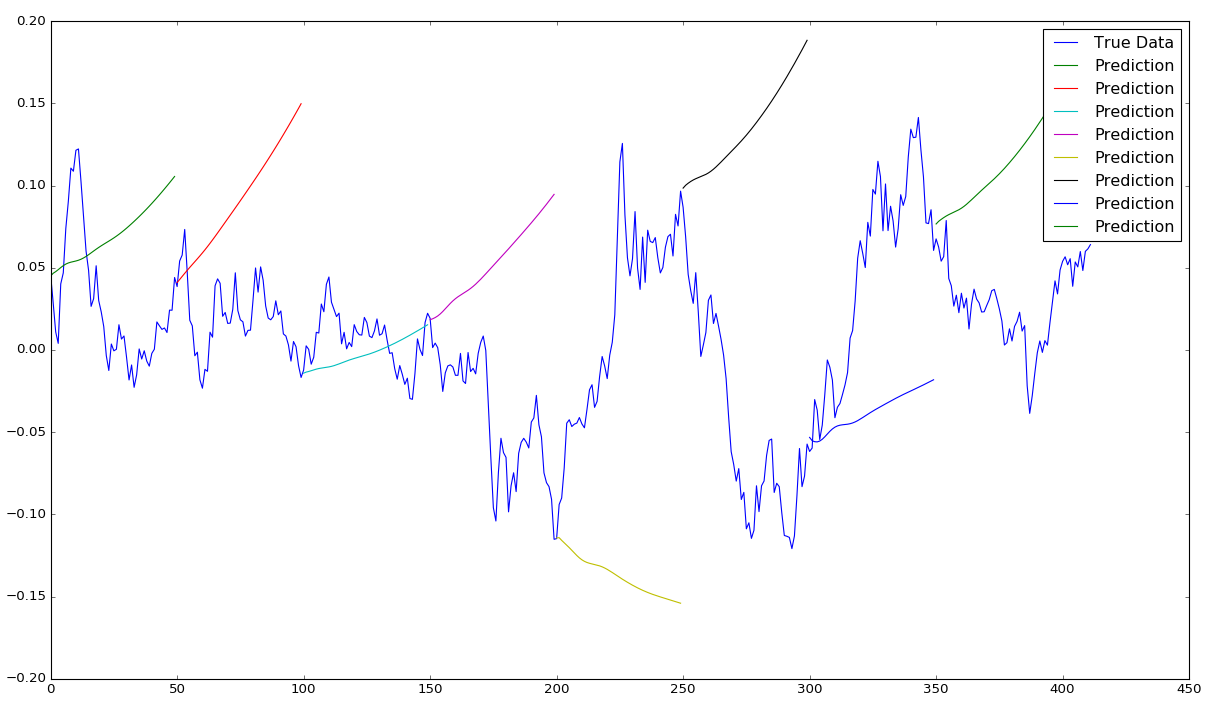epochs = 400, window size = 50, sequence shift = 50

LSTM的应用日益广泛，例如文本预测，AI智能聊天，自驾车等许多前沿领域。 希望本文能有助你开拓LSTM在时间序列中应用的视野。

【方向】
+ 关注